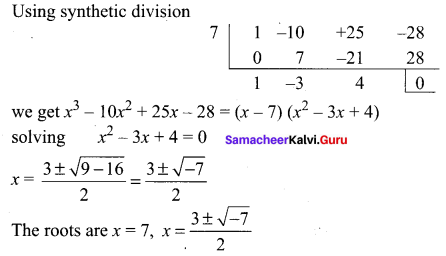# Samacheer Kalvi 11th Maths Solutions Chapter 2 Basic Algebra Ex 2.11

## Tamilnadu Samacheer Kalvi 11th Maths Solutions Chapter 2 Basic Algebra Ex 2.11

Question 1.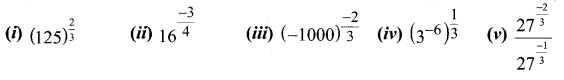Solution:
(i)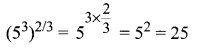(ii)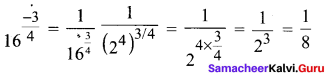(iii)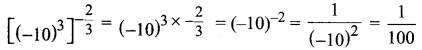(iv)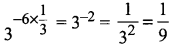(v)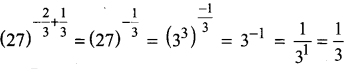Question 2.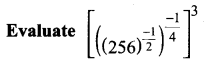Solution: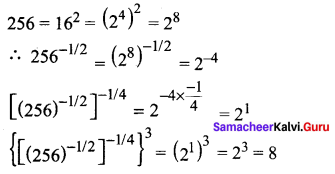Question 3.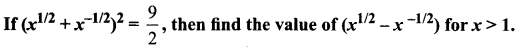Solution: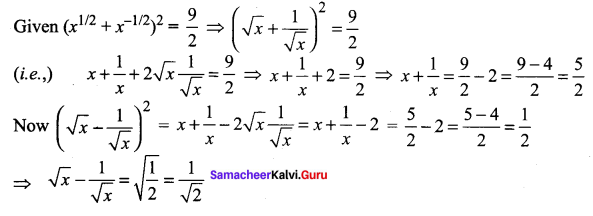Question 4.
Simplify and hence find the value of n : 32n92m-n/33n = 27
Solution: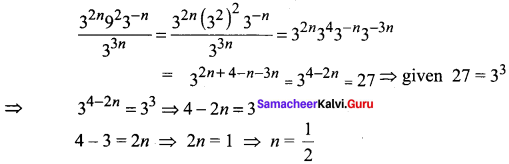Question 5.
Find the radius of the spherical tank whose volume is 32π/3 units.
Solution: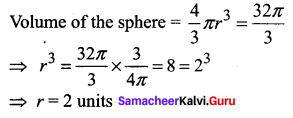Question 6.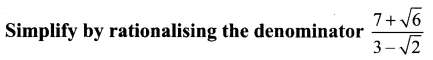Solution: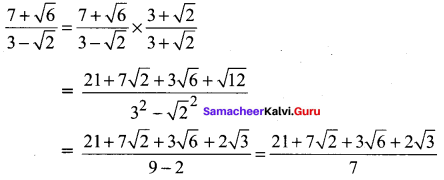Question 7.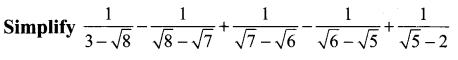Solution: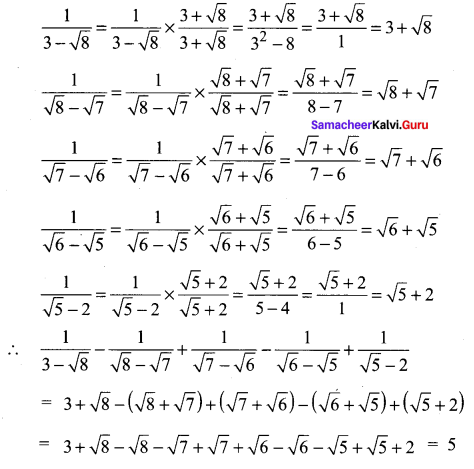Question 8.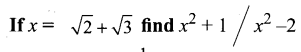Solution: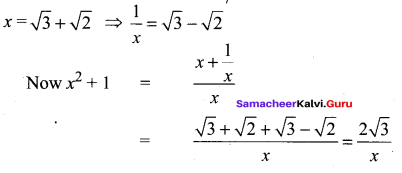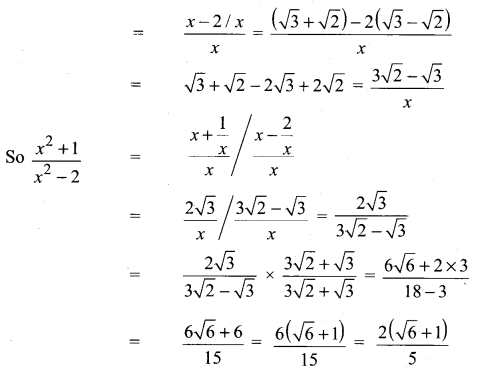### Samacheer Kalvi 11th Maths Solutions Chapter 2 Basic Algebra Ex 2.11 Additional Questions Solved

Question 1.
Simplify (343)2/3
Solution: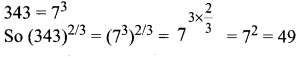Question 2.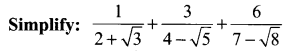Solution: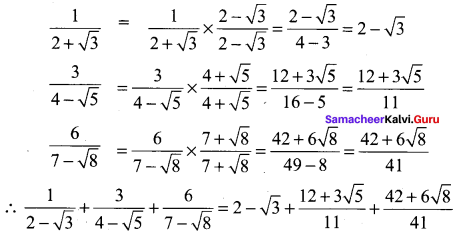Question 3.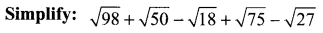Solution: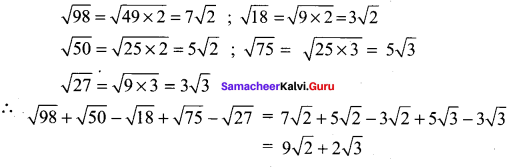Question 4.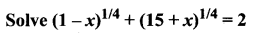Solution: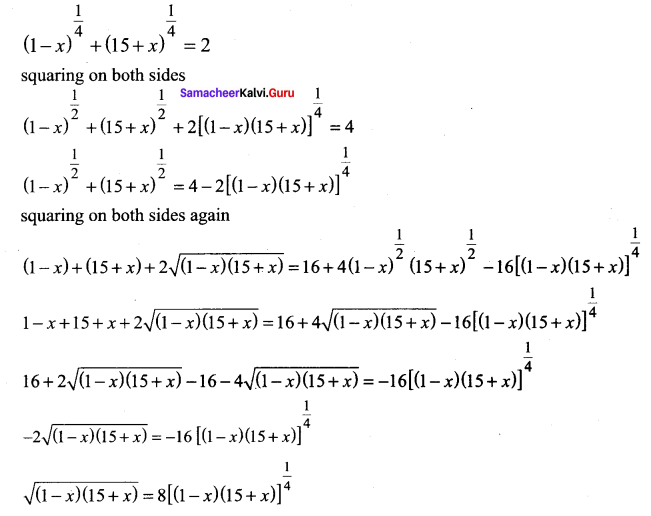squaring on bothsides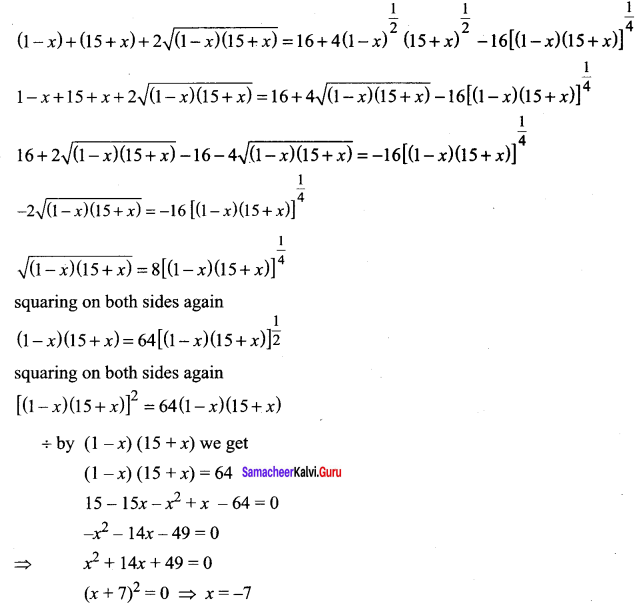Question 5.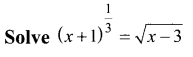Solution: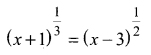L.C.M. of 2 and 3 is 6 & Raising to the power 6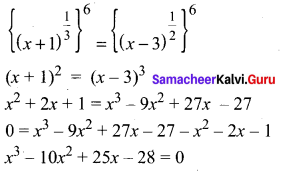since constant term is – 28
we can have a factor as (x ± 2) or (x ± 4) or (x ± 7)
By trial and error method we find that (x – 7) is a factor